American Journal of Optics and Photonics
Volume 3, Issue 5, October 2015, Pages: 114-117

Analysis of Near System in Terms of Image Retrieval

Sumit Kumar Pandey1, Kunal Kumar2

1Dept.of Electrical Engineering, Jharkhand Rai University, Ranchi India

2Dept. of Computer Science, St. Xavier’s College, Maharo, Dumka, Jharkhand, India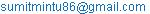(S. K. Pandey)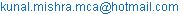(K. Kumar)

Sumit Kumar Pandey, Kunal Kumar. Analysis of Near System in Terms of Image Retrieval. American Journal of Optics and Photonics. Vol. 3, No. 5, 2015, pp. 114-117. doi: 10.11648/j.ajop.20150305.18

Abstract: In this paper, Near Set Evaluation and Recognition (NEAR) system has been evaluated and analyzed to retrieve perceptual information among images which can resemble human perception. NEAR System is a basic tool for image processing. Different image databases with varied category have been examined to determine the similarity among them. Work is preceded by partitioning an image into different objects. Nearness is performed on the basis of these objects. Work is done in version V3.0 of NEAR System. Calculation of equivalence classes, tolerance classes, segmentation evaluation, nearness among different images has been analyzed.

Keywords: Near Set Evaluation and Recognition (NEAR), Near Set Index (NSI)

1. Introduction

Near Set Evaluation And Recognition System (NEAR) System is a tool which works on feature-based approach to solve image correspondence problem i.e.to find the extent of similarity between images. Feature of images are the major concern in this tool which is applied during image segmentation evaluation. It works on the principle of near set theory. NEAR System is a framework used to find the perceptual nearness of different images. NEAR System is spurred by freely available software tool. NEAR system are having various versions as V1.0, V2.0, V3.0. The latest version of the NEAR system i.e. NEAR SYSTEM V3.0 includes region-of-interest, image neighbourhood analysis, topological structures of digital images. Various distance functions are also included to measure the degree of nearness or apartness among images. This system is coded in C++ and supports Multiple Document Interface (MDI) to enable the user to work with multiple processing tasks at the same time. Each image is divided into different sub images called as objects which are the basic operating part of NEAR System. By determining probe functions, feature values of different sub images or objects are created. Probe functions are the image processing functions applied on sub images . NEAR System provides the facility of adding new probe functions and new processing tasks .NEAR System is focused to perform the major tasks as to reveal equivalence and tolerance classes for an image, produce segmentation evaluation, measure the nearness among images, determine the nearness of neighborhoods of points in pairs of regions of interest (ROIs), display the locations of equivalence class sub images for a selected feature, perform Content-based image retrieval, display the output of processing an image using individual probe functions.

2. Calculative Works

All calculative works have been based on the division of an image into different sub images.

2.1. Calculation of Equivalence Class in an Image

Equivalence class can be defined as a set of objects satisfying perceptual indiscernibility relation , i.e. for a given image X, it calculates X/B, where the objects are sub images of X.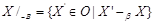eqn. 1

Here, normative indiscernibility relation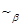can be calculated as:=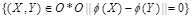eqn. 2

Here, <O, F> is a perceptual system and X, Y O .In this paper, an image taken from  database where the image is category of bus, the sub image size is taken as 20 with the number of features(probe functions)1 and the feature name is average grey.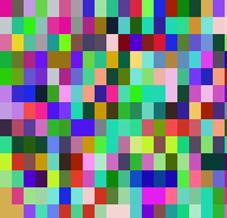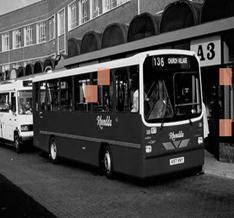Fig. 1(a). Original Image. Fig. 1(b). Equivalence class. Fig.1(c). Colored equivalence classes for sub image located at (x, y): (150, 115).

2.2. Calculation of Tolerance Classes

Tolerance classes is calculated using the Perceptual Tolerance i.e., for a given image X it find,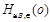, where the objects are subimages of X.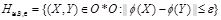eqn. 3

Tolerance class calculation of the image taken from has started by taking the window(sub image) size 20, epsilon value 0.010000 with one probe function as Average Grey.

In fig. 2(b), tolerance class has been generated after drafting the sub images of image 2(a) with sub image colour as black. Cross mark in fig. 2(b) indicates the tolerance classes for subimage located at (x,y): (243,144).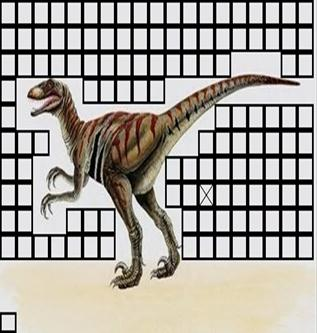Fig. 2(a). Original Image. Fig. 2(b). Tolerance class of original image.

2.3. Segmentation Evaluation

Two different images of elephant taken from  to perform image segmentation initiated by equivalence class calculation with widow (sub image) size 20 and Average G as one of the feature. Different color code in fig. 3(a) and 3(b) denotes different equivalence classes. Erode is performed by setting different Erode Near Set Parameters and the calculated Near Set Index (NSI) value is 5.7357. NSI is a metric based method which performs image segmentation without comparison to ground truth images.Erosion is a syntactic operation used to reduce the foreground area. It results in reduced foregroundfrom its outer edges to its inward area .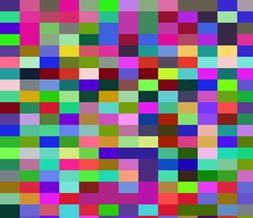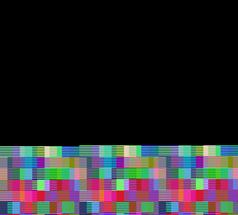Fig. 3(a). Quotient set image. Fig. 3(b). Segmentation image.

2.4. Nearness Among Images

NEAR System facilitates nearness measurement between either a pair of images or between a query image with directory of images. Fig. 4 is demonstrating the nearness between two different images of flower taken from  with epsilon value 0.01, sub image size 20 and Pal Entropy as probe function.Calculated result gives the value of tNM as 0.4102, tHD as 0.9997 and tHM as 0.9115 between two different images. Image entropy is the quantity or amount of information which must be coded for by a compression algorithm

Pal’s entropy can be calculated as: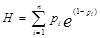eqn. 4

The gain in information from an event can be calculated as

∆I (pi ) = e(1−pi )

Entropy can be regarded as measure of gain of information from a single message.Gain of information is proportional to the probability of receiving the message.Assume that the probability of receiving a message i of n total messages is pi.Fig. 4(a). Image 1. Fig. 4(b). Image 2.

3. Results and Discussion

The time required to determined tolerance classes is high in the previous works. In this work the computation time has been reduced satisfactorily with more accurate result.Although the work has been done by CPU, implementing Graphics Processing Units (GPUs) increased the performance but overall time is reduced satisfactorily.

References

1. C. Henry and J. F. Peters, "Near set Evaluation And Recognition (NEAR) System",UM CI Laboratory Technical Report, Number TR-2009-015, p.no:1-18, 2009.
2. Database URL: http://wang.ist.psu.edu/docs/related/.
3. C. J. Henry, "Near Sets: Theory and Applications," Ph.D. Thesis, University of Winnipeg, Canada, 2010.
4. N. Jawas and N. Suciati, "Image Inpainting using Erosion and Dilation Operation", International Journal of Advanced Science and Technology, vol. 51, pp. 127-134, 2013.
5. C. Henry and J. F. Peters, "Near set index in an objective image segmentation evaluation Framework", Geobia, 2008–Pixels, Objects, Intelligence: GEOgraphic Object Based Image Analysis for the 21st Century, University of Calgary, Alberta, Canada, 6-7 Aug, 2008.
6. C. Henry and J. F. Peters, "Near set Evaluation And Recognition (NEAR) System V3.0", UM CI Laboratory Technical Report, Number TR-2012-019, January 9, 2012.

 Contents 1. 2. 2.1. 2.2. 2.3. 2.4. 3.
Article ToolsAbstractPDF(264K)